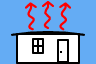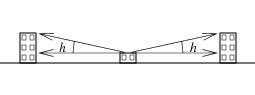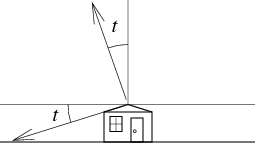http://people.csail.mit.edu/jaffer/SimRoof/NRT

With the assumption that the roof is at ambient temperature, only radiation needs to be considered when simulating the roof. SimRoof runs a stateless simulation driven from Typical Meteorological Year (TMY3) files containing hourly quantities for meteorological variables for locations throughout the United States, Puerto Rico, Virgin Islands, and Guam. Locations in Hawaii, Puerto Rico, Virgin Islands, and Guam are tropical.

99% of the sun's radiance is at wavelengths shorter than 4 µm; while 99% of a 25°C blackbody's radiance is at wavelengths longer than 4.8 µm. So we can consider solar and thermal radiance separately. The three quantities to compute for a prototypical square meter of roof are:

Solar Absorption
The direct and indirect solar radiation. Most of this radiation is at visible wavelengths.
The blackbody infrared radiation emitted by the roof.
This is the difficult part. Thermal infrared radiation is absorbed and re-emitted by water vapor at elevations throughout the troposphere. The spectrum of the re-emitted radiation depends on the temperature (and hence the altitude) of the water vapor emitting it.

More problematic may be emission and scattering by clouds. The sections Weather, Cloudless Sky, and Cloudy Sky discuss these models.

The specifics of these quantities are summarized in: Solar Absorption, Upward Thermal Radiation, and Downward Thermal Radiation.

Kirchhoff's Law of Thermal Radiation dictates that in every band, a body must emit the same proportion of thermal radiation as it absorbs. The Infrared Scattering page establishes that thermal-infrared radiation is not significantly scattered by clear air. Without scattering (or reflection), the correspondence between absorption and emission is of interest because it allows the use of atmospheric transmission data to calculate emissivity of cloudless atmosphere: That portion of mid- and far-infrared radiation which isn't transmitted is absorbed.

The Infrared Scattering by Water Clouds page argues that water and ice clouds can be treated as (opaque) blackbodies. The infrared transparency of the atmosphere between the ground and cloud-base is no different than that for cloudless sky. The cloud-base is modeled as the remaining moisture in a layer of liquid at the cloud-base height.

In the tropics, ice clouds occur at high altitudes. They will play a role only if there are not opaque clouds below them. Because high ice clouds are at cold temperatures (as estimated by the lapse-rate), treating them as cloudless sky will not introduce large errors.

## Simulation Inputs

The following tables list the computational inputs for simulating solar-absorption, upward-thermal-radiation, and downward-thermal-radiation. Each table has three columns: Lambertian roofs, roofs with angle-dependent emissivity and absorption, and roofs with angle-dependent and wavelength-dependent emissivity and absorption.

The angle-dependence is the angle from zenith. Angle-dependent optical functions allow modeling of the aperture-restriction discussed in Optics for Passive Radiative Cooling.Angle-dependent optical functions can model when buildings or terrain obstruct the horizon. This can be done in a manner analogous to the way clouds are treated: for each angle near the horizon, the obstruction percentage of the incoming radiation comes from a blackbody at a temperature at or above ambient through the average distance to the obstructions. During the day, the temperature of obstructed horizons is greater than ambient due to solar heating. Introduced is a solar-temperature-gain (stg) parameter which is the temperature increment (°C) per 1000 W of TMY3 Global-Horizontal-Irradiance (ghi). For an angle h above the horizon (π/2−h from the zenith), the solid angle to the horizontal plane is π·sin2(h)=(π/2) (1−cos(h)). For a given solid angle γ, the h having the same solid angle is cos-1(1−(2/π)·γ).If the roof has a small tilt t, then the airmass over most of the hemisphere is not much different than for a horizontal roof. The difference is that a spherical wedge between the plane of the roof and the horizontal plane receives thermal radiation from the ground or buildings. The solid angle subtended by tilt angle t is 2t (radians).

Lambertian RoofAngle DependentAngle and Wavelength Dependent
The simplest configuration is a horizontal, Lambertian surface under a full hemisphere of unobstructed sky. Aperture restricted to a cone around the zenith; also handles obstructed horizons and small roof tilts (less than 15°). Spectral optical function, horizon obstructions, and daylight spectra must be compiled into the NIRT array, making it specific to a single configuration.

### Solar Absorption

Lambertian RoofAngle DependentAngle and Wavelength Dependent
Roof Solar Absorption
The fraction of solar power absorbed; between 0 and 1.
Roof Aperture Cone Angle Roof Solar Optical Absorption Function
The angle centered on the zenith of the cone from which solar radiation is absorbed. The angle is between 0° and 180°. Function of solar-elevation (angle) and wavelength.
Total amount of direct and diffuse solar radiation received on a horizontal surface during the 60-minute period. Amount of solar radiation (modeled) received in a collimated beam on a surface normal to the sun during the 60-minute period.
Amount of solar radiation received from the sky (excluding the solar disk) on a horizontal surface during the 60-minute period.
Solar-Elevation
Computed from:
TMY3 Time
Hour of day

TMY3 Date
Day of year between 0 and 365.

Site Latitude
Between −90° and +90°.
Tropical latitudes are between −23.5° and +23.5°.

Lambertian RoofAngle DependentAngle and Wavelength Dependent
Roof Emissivity
The fraction of possible blackbody power radiated; between 0 and 1.
Roof Aperture Cone Angle Emissivity Function
The angle centered on the zenith of the cone from which thermal radiation is absorbed. The angle is between 0° and 180°. function of angle and wavelength.
TMY3 Dry-bulb
Ambient temperature in °C.

Lambertian RoofAngle DependentAngle and Wavelength Dependent
Roof Emissivity
The fraction of possible blackbody power radiated; between 0 and 1.
Roof Aperture Cone Angle Emissivity Function
The angle centered on the zenith of the cone from which thermal radiation is absorbed. The angle is between 0° and 180°. function of angle and wavelength.
TMY3 Dry-bulb
Ambient temperature in °C.
Ignoring outlying negative temperatures from 3 sites, the tropical TMY3 range is 12.8°C to 36.6°C.
TMY3 Opaque sky cover
Amount of sky dome covered by clouds or obscuring phenomena that prevent observing the sky or higher cloud layers at the time indicated.
Range is 0% to 100% at 20% resolution.
TMY3 Ceiling height
Height of the cloud base above local terrain.
Tropical TMY3 range is 0 m to 21999 m.
TMY3 Precipitable water
The total precipitable water contained in a column of unit cross section extending from the earth's surface to the top of the atmosphere.
Tropical TMY3 range is 11 mm to 65 mm.
TMY3 Atmospheric Pressure
Station pressure at the time indicated; 101.325 kPa in the International Standard Atmosphere.
Tropical TMY3 range is 95800 Pa to 103600 Pa.
Atmospheric Transparency
Valued 0 to 1, a function of precipitable-water and wavelength.
Z
Height of the tropopause (top of the troposphere); 16 km in the tropics; 11 km in ISA.
L
Troposphere lapse-rate; 6.5 K/km (ISA).

All the inputs for computing upward-thermal-radiation are also inputs for computing downward-thermal-radiation; and computing upward-thermal-radiation is an order of magnitude simpler than downward-thermal-radiation. So the upward and downward thermal radiation computations can be combined.

troposphere.pdf gives the mathematical details of the thermal radiation calculation.

## Simulation Strategy

There are 8760 hours in a (typical meteorological) year. Computing the thermal radiation balance separately for each hour is needlessly wasteful of computational resources. Instead, compute and save the thermal-radiation flow at a grid of points in the space spanned by the tropical ranges of
• ceiling-height,
• dry-bulb temperature,
• precipitable-water, and
• atmospheric-pressure.
Then interpolate between these values to compute the hourly thermal-radiation.

For Lambertian roofs (described in Simulation Inputs), a single table can be used to simulate any TMY3 (whose values are within its range). For rotational-symmetry cases a single table which stores values for each ray-angle can be used, 5850 values in the example below. For the general-case (wavelength-dependent emissivity), a table must be calculated for each emissivity-function. But that table is valid for any TMY3 (whose values are within its range).

The CoolRoof page shows the results of running the tropical model with Guam TMY3 data.